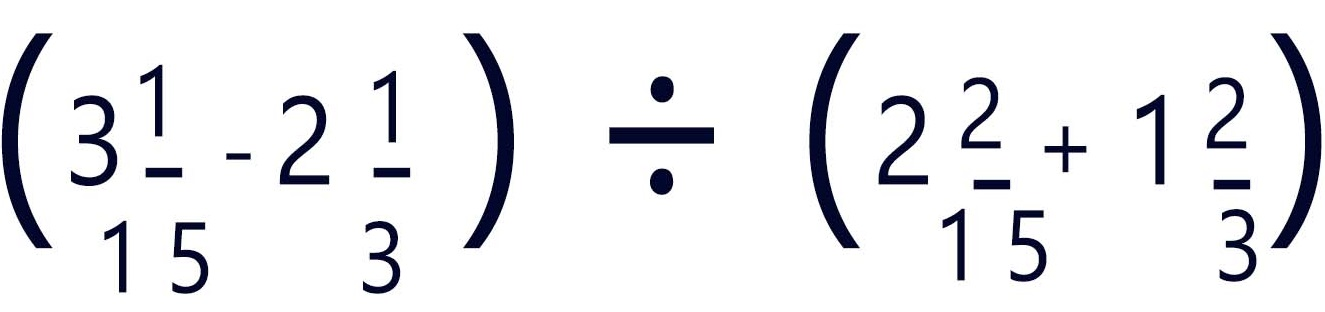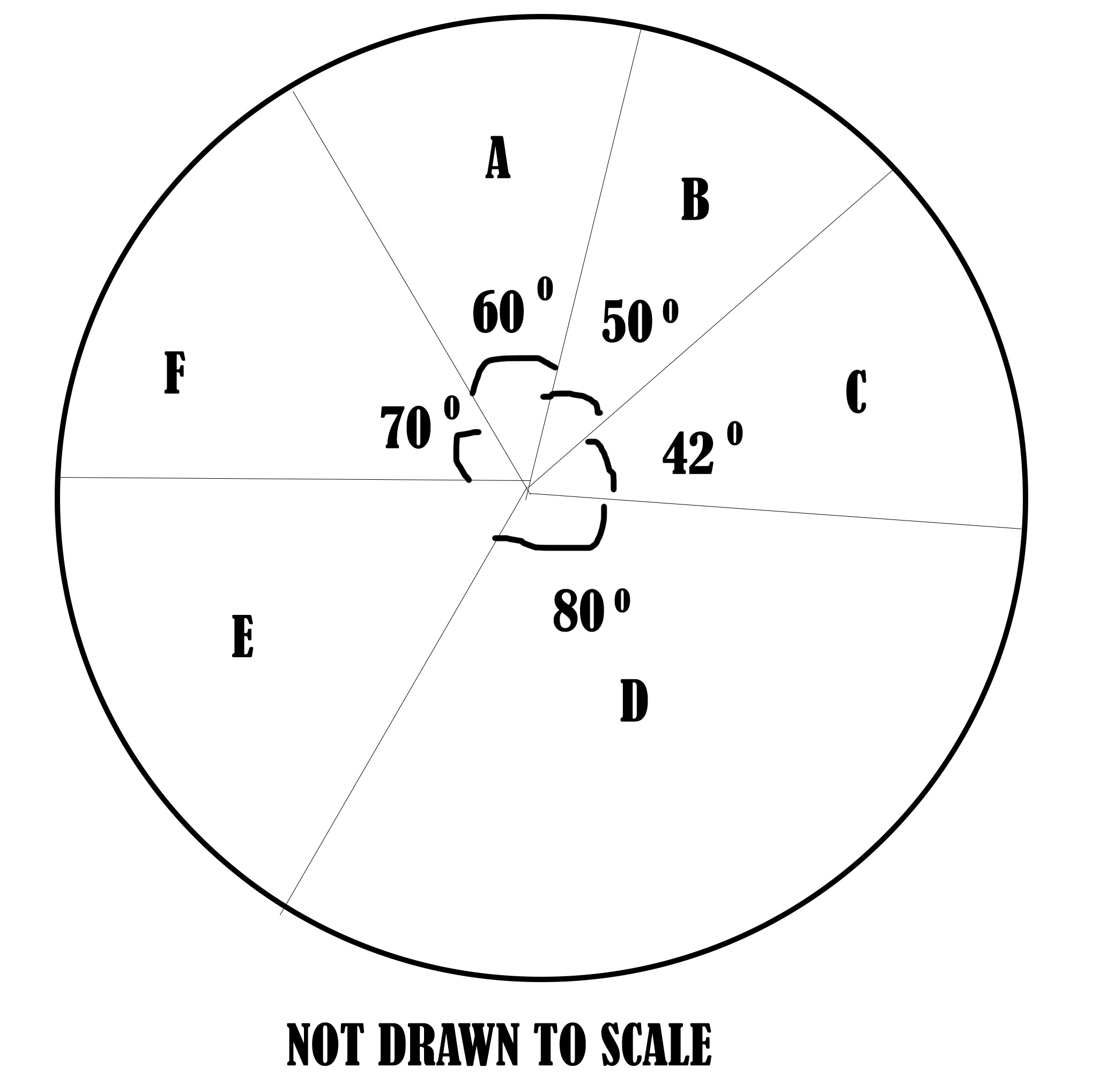Kuulchat

Online Education Resource Portal, its all freeJ.H.S MATHEMATICS

Question 1

a)

Given that P,R and W are sets such that,P and R are subsets of W.

W={natural numbers greater or equal 3 but less than 21}

P={multiples of 3}

R={factors of 18}

i. Find the elements of sets P,R and W

ii. Find
α P'
β (PR)'

b)

Simplifyc)

In a school, ⅙ of the students speak French, ⅔ of the school speak Ga and the rest speak Twi. If 60 students speak Twi, how many students are in the school?

Question 2

The pie chart below shows the distribution of textbooks to six classes A,B,C,D,E and F in a school(i)

If class D was given 720 textbooks, how many textbooks were distributed to each of the remaining classes?

(ii)

What is the average number of textbooks distributed to the classes?

(iii)

How many classes had less than the average number of textbooks distributed?